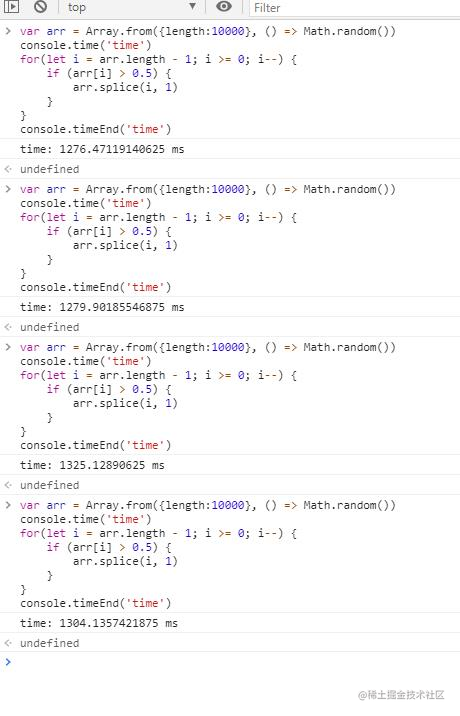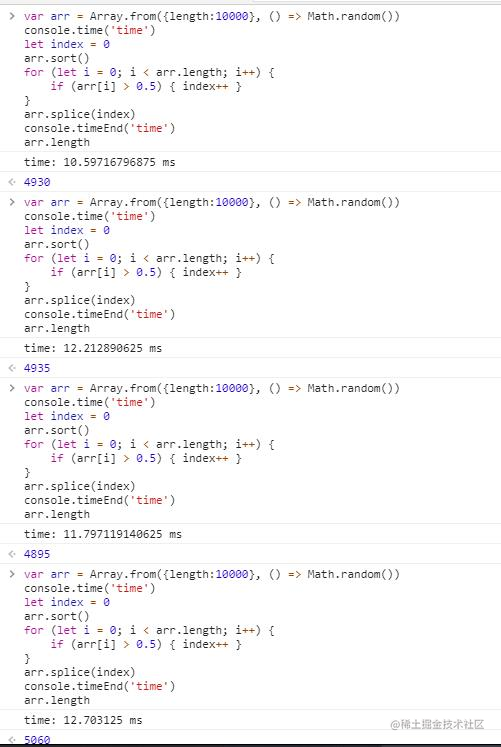# 场景

``````class DataArr extends Array {
constructor(data, key){
super()
this.dataMap = {}
this.dataKey = key
this.dataWhichBeFiltered = []
}
initData () {
this.forEach((item, index) => {
this.initItem(item, {
index
})
})
}
initItem (item, opt) {
this.dataMap[item[this.dataKey]] = item
item._index = opt.index
}
filter () {}
resetFilter () {}
}

// usage
const data = new DataArr(arr)

data.filter(item => item.a === 1)
data.resetFilter()

# 过程

``````filter (fn) {
for (let i = this.length - 1; i >= 0 ;i--) {
const item = this[i]
if (!!fn(item)) {
const delItem = this.splice(i, 1)
this.dataWhichBeFiltered.push(delItem)
}
}
}

``````filter (fn) {
let index = 0
for (let i = this.length - 1; i >= 0 ;i--) {
const item = this[i]
if (!!fn(item)) {
item.willBeFiltered = 1
index ++
}
else item.willBeFiltered = 0
}
this.sort((a, b) => b.willBeFiltered - a.willBeFiltered)
this.dataWhichBeFiltered = this.splice(i)
}# 原由

splice 的缓慢在于数组的插入移除操作不像链表，只需要切除要移除的节点，再把 prev 节点和 next 节点连接就完成了。

``````数组的变化（移除前-移除后）
0 => 1          0 => 1
1 => 2          1 => 2
2 => 3          2 => 3
3 => 4          3 => 4
4 => 5    =>    4 => 6
5 => 6    =>    5 => 7
6 => 7    =>    6 => 8
7 => 8    =>    7 => 9
8 => 9    =>    8 => 10
9 => 10

# tips

``````    this.dataWhichBeFiltered.forEach(data => this.push(data))
this.sort((a, b) => a._index - b._index)

arr.push(element1, ..., elementN)

# 总结

Hello 这里是 Roxz， 欢迎给我的文章点赞~我会在精神上默默感谢你的鼓励。